# raypl

Path loss and phase change for RF propagation ray

## Syntax

``[pl,phase] = raypl(ray)``
``[pl,phase] = raypl(ray,Name,Value)``

## Description

example

````[pl,phase] = raypl(ray)` returns the path loss `pl` in dB and phase shift `phase` in radians for the RF propagation ray `ray`. The function calculates the path loss and phase shift using free space loss and reflection loss derived from the propagation path, reflection materials, and antenna polarizations.By default, `raypl` assumes the antennas are unpolarized. You can polarize the antennas by specifying the `TransmitterPolarization` and `ReceiverPolarization` name-value arguments.For more information about the path loss computations, see Path Loss Computation.```

example

````[pl,phase] = raypl(ray,Name,Value)` specifies options using name-value arguments. For example, `"ReflectionMaterials","brick"` specifies the reflection material as brick.```

## Examples

collapse all

Change the reflection materials and frequency for a ray and reevaluate the path loss and phase shift.

Launch Site Viewer with buildings in Hong Kong. For more information about the osm file, see . Specify transmitter and receiver sites.

```viewer = siteviewer("Buildings","hongkong.osm"); tx = txsite("Latitude",22.2789,"Longitude",114.1625, ... "AntennaHeight",10,"TransmitterPower",5, ... "TransmitterFrequency",28e9); rx = rxsite("Latitude",22.2799,"Longitude",114.1617, ... "AntennaHeight",1);```

Perform ray tracing between the sites.

```pm = propagationModel("raytracing", ... "Method","image", ... "MaxNumReflections",2); rays = raytrace(tx,rx,pm);```

Find the first ray with 2-order reflections from the result. Display the ray characteristics. Plot the ray to see the ray reflect off two buildings.

`ray = rays{1}(find([rays{1}.NumInteractions] == 2,1))`
```ray = Ray with properties: PathSpecification: 'Locations' CoordinateSystem: 'Geographic' TransmitterLocation: [3×1 double] ReceiverLocation: [3×1 double] LineOfSight: 0 Interactions: [1×2 struct] Frequency: 2.8000e+10 PathLossSource: 'Custom' PathLoss: 121.8178 PhaseShift: 4.5601 Read-only properties: PropagationDelay: 8.3060e-07 PropagationDistance: 249.0068 AngleOfDeparture: [2×1 double] AngleOfArrival: [2×1 double] NumInteractions: 2 ```
`plot(ray)`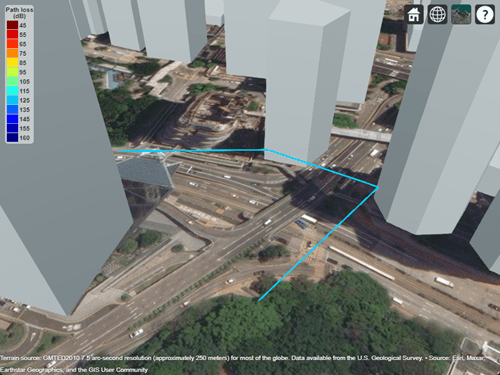By default, all buildings have concrete building material electrical characteristics. Change the material to metal for the second reflection and re-evaluate path loss. Use the `raypl` function to reevaluate the pathloss for the ray. Display the ray path to compare the change in path loss. Replot to show the slight change in color due to the path loss change of the ray.

```[ray.PathLoss,ray.PhaseShift] = raypl(ray, ... "ReflectionMaterials",["concrete","metal"])```
```ray = Ray with properties: PathSpecification: 'Locations' CoordinateSystem: 'Geographic' TransmitterLocation: [3×1 double] ReceiverLocation: [3×1 double] LineOfSight: 0 Interactions: [1×2 struct] Frequency: 2.8000e+10 PathLossSource: 'Custom' PathLoss: 117.1214 PhaseShift: 4.5601 Read-only properties: PropagationDelay: 8.3060e-07 PropagationDistance: 249.0068 AngleOfDeparture: [2×1 double] AngleOfArrival: [2×1 double] NumInteractions: 2 ```
```ray = Ray with properties: PathSpecification: 'Locations' CoordinateSystem: 'Geographic' TransmitterLocation: [3×1 double] ReceiverLocation: [3×1 double] LineOfSight: 0 Interactions: [1×2 struct] Frequency: 2.8000e+10 PathLossSource: 'Custom' PathLoss: 117.1214 PhaseShift: 4.5601 Read-only properties: PropagationDelay: 8.3060e-07 PropagationDistance: 249.0068 AngleOfDeparture: [2×1 double] AngleOfArrival: [2×1 double] NumInteractions: 2 ```
`plot(ray)`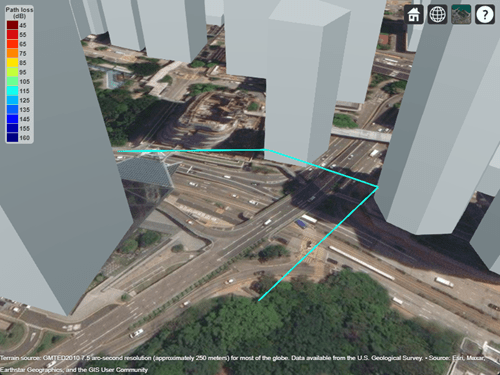Change the frequency and reevaluate the path loss and phase shift. Plot the ray again and observe the obvious color change.

```ray.Frequency = 2e9; [ray.PathLoss,ray.PhaseShift] = raypl(ray, ... "ReflectionMaterials",["concrete","metal"]); plot(ray)```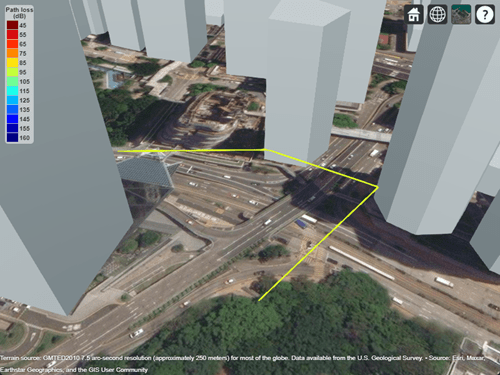Appendix

## Input Arguments

collapse all

RF propagation ray, specified as one `comm.Ray` object. The `PathSpecification` property of the object must be `"Locations"`.

Data Types: `comm.Ray`

### Name-Value Arguments

Specify optional pairs of arguments as `Name1=Value1,...,NameN=ValueN`, where `Name` is the argument name and `Value` is the corresponding value. Name-value arguments must appear after other arguments, but the order of the pairs does not matter.

Before R2021a, use commas to separate each name and value, and enclose `Name` in quotes.

Example: `raypl(ray,"TransmitterPolarization","H","ReceiverPolarization","H")`, specifies the horizontal polarizations for the transmit and receive antennas for `ray`.

Reflection materials for a non-line-of-sight (NLOS) ray, specified as a string scalar, a 1-by-NR string vector, a character vector, a 1-by-NR cell array of character vectors, a 2-by-1 numeric vector, or a 2-by-NR numeric matrix. NR is the number of reflections stored in the `NumReflections` property of `ray`.

When you specify one reflection material, the reflection material applies to all the reflections. When you specify multiple reflection materials, each material applies to the associated reflection point in `ray`.

• To use predefined reflection materials, specify `ReflectionMaterials` as a string scalar, a character vector, a string vector, or a cell array of character vectors. Specify each reflection material as one of these options: `"concrete"`, `"brick"`, `"wood"`, `"glass"`, `"plasterboard"`, `"ceiling-board"`, `"chipboard"`, `"floorboard"`, `"metal"`, `"water"`, `"vegetation"`, `"loam"`, or `"perfect-reflector"`.

• To use custom reflection materials, specify a 2-by-1 numeric vector or a 2-by-NR numeric matrix. Each column is of the form `[rp; cv]`, where `rp` is the relative permittivity and `cv` is the conductivity.

For more information, see ITU Permittivity and Conductivity Values for Common Materials.

Example: `"ReflectionMaterials",["concrete","water"]`, specifies that a ray with two reflections uses the electrical characteristics of concrete at the first reflection point and water at the second reflection point.

Data Types: `string` | `char` | `double`

Transmit antenna polarization type, specified as one of these values:

• `"none"` — Unpolarized

• `"V"` — Linearly polarized in the vertical (θ) direction

• `"H"` — Linearly polarized in the horizontal (φ) direction

• `"LHCP"` — Left-hand circular polarized

• `"RHCP"` — Right-hand circular polarized

• A normalized 2-by-1 Jones vector (also called a polarization matrix) of the form `[H;V]`, where `H` is the horizontal component and `V` is the vertical component.

For more information about polarization types and Jones vectors, see Jones Vector Notation.

Example: `"TransmitterPolarization","RHCP"` specifies right-hand circular polarization for the transmit antenna.

Data Types: `double` | `char` | `string`

Receive antenna polarization type, specified as one of these values:

• `"none"` — Unpolarized

• `"V"` — Linearly polarized in the vertical (θ) direction

• `"H"` — Linearly polarized in the horizontal (φ) direction

• `"LHCP"` — Left-hand circular polarized

• `"RHCP"` — Right-hand circular polarized

• A normalized 2-by-1 Jones vector (also called a polarization matrix) of the form `[H;V]`, where `H` is the horizontal component and `V` is the vertical component.

For more information about polarization types and Jones vectors, see Jones Vector Notation.

Example: `"ReceiverPolarization",[1;0]` specifies horizontal polarization for the receive antenna by using Jones vector notation.

Data Types: `double` | `char` | `string`

Orientation of the transmit antenna axes, specified as a 3-by-3 unitary matrix indicating the rotation from the transmitter local coordinate system (LCS) into the global coordinate system (GCS). When the `CoordinateSystem` property of the `comm.Ray` is set to `"Geographic"`, the GCS orientation is the local East-North-Up (ENU) coordinate system at transmitter. For more information, see Coordinate System Orientation.

Example: `"TransmitterAxes",eye(3)`, specifies that the local coordinate system for the transmitter axes is aligned with the global coordinate system. This is the default orientation.

Data Types: `double`

Orientation of the receive antenna axes, specified as a 3-by-3 unitary matrix indicating the rotation from the receiver local coordinate system (LCS) into the global coordinate system (GCS). The GCS orientation is the local East-North-Up (ENU) coordinate system at receiver when the .`CoordinateSystem` property of the `comm.Ray` is set to `"Geographic"`. For more information, see Coordinate System Orientation.

Example: `"ReceiverAxes",[0 -1 0; 1 0 0; 0 0 1]`, specifies a 90° rotation around the z-axis of the local receiver coordinate system with respect to the global coordinate system.

Data Types: `double`

## Output Arguments

collapse all

Path loss in dB, returned as a nonnegative scalar.

Data Types: `double`

Phase shift in radians, returned as a scalar in the range [–π, π] radians. The argument uses the e-iωt time convention.

Data Types: `double`

collapse all

### ITU Permittivity and Conductivity Values for Common Materials

ITU-R P.2040-1  and ITU-R P.527-5  present methods, equations, and values used to calculate real relative permittivity, conductivity, and complex relative permittivity for the common materials.

### Coordinate System Orientation

This image shows the orientation of the electromagnetic fields in the global coordinate system (GCS) and the local coordinate systems of the transmitter and receiver.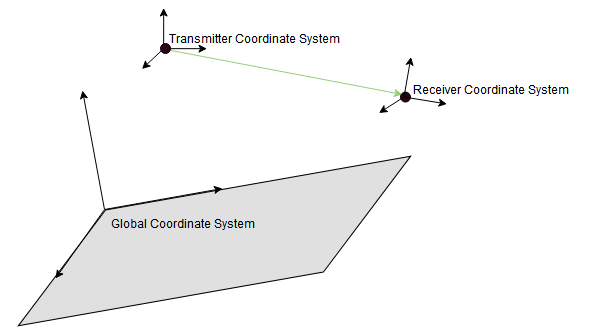When the `CoordinateSystem` property of the `comm.Ray` is set to `"Geographic"`, the GCS orientation is the local East-North-Up (ENU) coordinate system at observer. The path loss computation accounts for the round-earth differences between ENU coordinates at the transmitter and receiver.

### Path Loss Computation

The ray tracing model used by the `raypl` function calculates reflection losses by tracking the horizontal and vertical polarizations of signals through the propagation path. Total power loss is the sum of free space loss and reflection loss.

This image shows a reflection path from a transmitter site tx to a receiver site rx.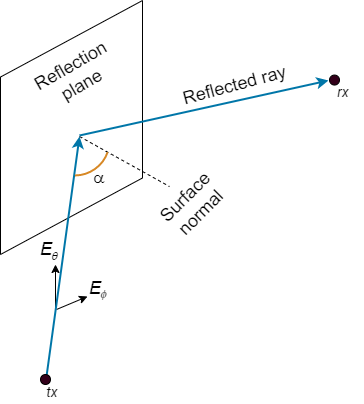The model determines polarization and reflection loss using these steps.

1. Track the propagation of the ray in 3-D space by calculating the propagation matrix P. The matrix is a repeating product, where i is the number of reflection points.

`$P=\prod _{i}{P}_{i}$`

For each reflection, calculate Pi by transforming the global coordinates of the incident electromagnetic field into the local coordinates of the reflection plane, multiplying the result by a reflection coefficient matrix, and transforming the coordinates back into the original global coordinate system . The equations for Pi and P0 are:

`${P}_{i}=\begin{array}{ccc}{\left[\begin{array}{ccc}{s}_{out}& {p}_{out}& {k}_{out}\end{array}\right]}_{i}& {\left[\begin{array}{ccc}{R}_{V}\left(\alpha \right)& 0& 0\\ 0& {R}_{H}\left(\alpha \right)& 0\\ 0& 0& 1\end{array}\right]}_{i}& {\left[\begin{array}{ccc}{s}_{in}& {p}_{in}& {k}_{in}\end{array}\right]}_{i}^{-1}\end{array}$`

`${P}_{0}=\left[\begin{array}{ccc}1& 0& 0\\ 0& 1& 0\\ 0& 0& 1\end{array}\right]$`

where:

• s, p, and k form a basis for the plane of incidence (the plane created by the incident ray and the surface normal of the reflection plane). s and p are perpendicular and parallel, respectively, to the plane of incidence.

• kin and kout are the directions (in global coordinates) of the incident and exiting rays, respectively.

• sin and sout are the directions (in global coordinates) of the horizontal polarizations for the incident and exiting rays, respectively.

• pin and pout are the directions (in global coordinates) of the vertical polarizations for the incident and exiting rays, respectively.

• RH and RV are the Fresnel reflection coefficients for the horizontal and vertical polarizations, respectively. α is the incident angle of the ray and εr is the complex relative permittivity of the material.

${R}_{H}\left(\alpha \right)=\frac{\mathrm{cos}\left(\alpha \right)-\sqrt{\left({\epsilon }_{r}-{\mathrm{sin}}^{2}\left(\alpha \right)\right)/{\epsilon }_{r}{}^{2}}}{\mathrm{cos}\left(\alpha \right)+\sqrt{\left({\epsilon }_{r}-{\mathrm{sin}}^{2}\left(\alpha \right)\right)/{\epsilon }_{r}{}^{2}}}$

${R}_{V}\left(\alpha \right)=\frac{\mathrm{cos}\left(\alpha \right)-\sqrt{{\epsilon }_{r}-{\mathrm{sin}}^{2}\left(\alpha \right)}}{\mathrm{cos}\left(\alpha \right)+\sqrt{{\epsilon }_{r}-{\mathrm{sin}}^{2}\left(\alpha \right)}}$

2. Project the propagation matrix P into a 2-by-2 polarization matrix R. The model rotates the coordinate systems for the transmitter and receiver so that they are in global coordinates.

`$R=\left[\begin{array}{cc}{H}_{in}\cdot {H}_{rx}& {V}_{in}\cdot {H}_{rx}\\ {H}_{in}\cdot {V}_{rx}& {V}_{in}\cdot {V}_{rx}\end{array}\right]$`
`${H}_{in}=P\left({V}_{tx}×{k}_{tx}\right)$`
`${V}_{in}=P{V}_{tx}$`

where:

• Hrx and Vrx are the directions (in global coordinates) of the horizontal (Eθ) and vertical (Eϕ) polarizations, respectively, for the receiver.

• Hin and Vin are the directions (in global coordinates) of the propagated horizontal and vertical polarizations, respectively.

• Vtx is the direction (in global coordinates) of the nominal vertical polarization for the ray departing the transmitter.

• ktx is the direction (in global coordinates) of the ray departing the transmitter.

3. Specify the normalized horizontal and vertical polarizations of the electric field at the transmitter and receiver by using the 2-by-1 Jones polarization vectors Jtx and Jrx, respectively. If either the transmitter or receiver are unpolarized, then the model assumes ${J}_{tx}={J}_{rx}=\frac{\sqrt{2}}{2}\left[\begin{array}{c}1\\ 1\end{array}\right]$.

4. Calculate the polarization and reflection loss IL by combining R, Jtx, and Jrx.

`$IL=-20{\mathrm{log}}_{10}|{J}_{rx}^{-1}R{J}_{tx}|$`

### Jones Vector Notation

For Jones vector notation, the raypl function describes signal polarization using Jones calculus.

The orthogonal components of Jones vectors are defined for Eθ and Eφ. This table shows the Jones vector corresponding to various antenna polarizations.

Antenna Polarization TypeCorresponding Jones Vector

Linear polarized in the θ direction

`$\left(\begin{array}{c}H\\ V\end{array}\right)=\left(\begin{array}{c}0\\ 1\end{array}\right)$`

Linear polarized in the φ direction

`$\left(\begin{array}{c}H\\ V\end{array}\right)=\left(\begin{array}{c}1\\ 0\end{array}\right)$`

Left-hand circular polarized (LHCP)

`$\left(\begin{array}{c}H\\ V\end{array}\right)=\frac{1}{\sqrt{2}}\left(\begin{array}{c}j\\ 1\end{array}\right)$`

Right-hand circular polarized (RHCP)

`$\left(\begin{array}{c}H\\ V\end{array}\right)=\frac{1}{\sqrt{2}}\left(\begin{array}{c}-j\\ 1\end{array}\right)$`

 Chipman, Russell A., Garam Young, and Wai Sze Tiffany Lam. "Fresnel Equations." In Polarized Light and Optical Systems. Optical Sciences and Applications of Light. Boca Raton: Taylor & Francis, CRC Press, 2019.

 International Telecommunications Union Radiocommunication Sector. Effects of building materials and structures on radiowave propagation above about 100MHz. Recommendation P.2040-1. ITU-R, approved July 29, 2015. https://www.itu.int/rec/R-REC-P.2040-1-201507-I/en.

 International Telecommunications Union Radiocommunication Sector. Attenuation by atmospheric gases. Recommendation P.676-11. ITU-R, approved September 30, 2016. https://www.itu.int/rec/R-REC-P.676-11-201609-S/en.